Courses

# Logical Effort Calculation of few Basic Logic Circuits Electrical Engineering (EE) Notes | EduRev

## Electrical Engineering (EE) : Logical Effort Calculation of few Basic Logic Circuits Electrical Engineering (EE) Notes | EduRev

The document Logical Effort Calculation of few Basic Logic Circuits Electrical Engineering (EE) Notes | EduRev is a part of Electrical Engineering (EE) category.
All you need of Electrical Engineering (EE) at this link: Electrical Engineering (EE)

Objectives

In this lecture you will learn the following

• Introduction
• Logical Effort of an Inverter
• Logical Effort of NAND Gate
• Logical Effort of NOR Gate
• Logical Effort of XOR Gate
• Logic Effort Calculation of few Mixed Circuits
• Delay Plot

22.1 Introduction

The method of logical effort is an easy way to estimate delay in a CMOS circuit. We can select the fastest candidate by comparing delay estimates of different logic structures. The method also specifies the proper number of logic stages on a path and the best transistor sizes for the logic gates. Because the method is easy to use, it is ideal for evaluating alternatives in the early stages of a design and provides a good staring point for more intricate optimizations. It is founded on a simple model of the delay through a single MOS logic gate. The model describes delays caused by the capacitive load that the logic gate drives and by the topology of the logic gate. Clearly as the load increases, the delay increases, but the delay also depends on the logic function of the gate. Inverters, the simplest logic gates, drive loads best and are often used as amplifiers to drive large capacitances. Logic gates that compute other functions require more transistors, some of which are connected in series, making them poorer than inverters at driving current. Thus a NAND gate has more delay than an inverter with similar transistor sizes that drives the same load. The method of logical effort quantifies these effects to simplify delay analysis for individual logic gates and multistage logic networks.

The method of logical effort is founded on a simple model of the delay through a single MOS logic gate. The model describes delay caused by the capacitive load that the logic gate drives. Certainly as the load increases the delay increases, but delay also depends on logical function of the gate. Invertors, the simplest logical gates, drive loads best and are often used as amplifiers to drive large capacitances. Logic gates that compute other functions require more transistors, some connected in series, making them poorer than inverters at driving currents. Thus NAND gate has more delay than inverter with similar transistor size and driving load. The method of logical effort qualifies these effects to simplify delay analysis for individual logic gates and multistage logic networks.

22.2 Logical Effort of an Inverter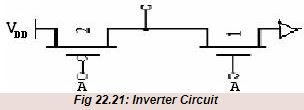The logical effort of an Inverter is defined to be unity.

22.3 Logical Effort of a NAND Gate

A NAND gate contain two NMOS (pull down) transistors in series and two PMOS (pull up)transistors as shown in fig 22.3)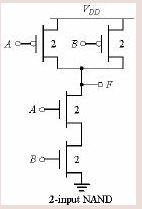Fig 22.3:2-input NAND

We have to size the transistors such that the gate has the same drive characteristics as an inverter with a pull down of width 1 and a pull up of width 2. Because the two pull down transistors are in series, each must have the twice the conductance of the inverter pull down transistor so that the series connection has a conductance equal to that of the inverter pull down transistor. Hence these two transistors should have twice the width compared to inverter pull down transistor. By contrast, each of the two pull up transistors in parallel need be only as large as the inverter pull up transistor to achieve the same drive as the reference inverter. So, the logical effort per input can be calculated as

g = (2+2)/ (1+2) = 4/3.
For 3 input NAND gate, g = (3+2)/ (1+2) =5/3
For n input NAND gate, g = (n+2)/ 3

22.4 Logical Effort of a NOR Gate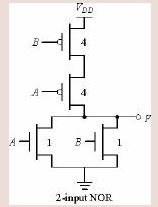Fig 22.4: 2-input NOR

A NOR gate contain two pull down transistors in parallel and two pull up transistors in series as shown in figure 22.4

Because the two pull up transistors are in series, each must have the twice the conductance of the inverter pull up transistor so that the series connection has a conductance equal to that of the inverter pull up transistor. Hence these two transistors should have twice the width compared to inverter pull down transistor. By contrast, each of the two pull down transistors in parallel need be only as large as the inverter pull down transistor to achieve the same drive as the reference inverter. So, the logical effort per input can be calculated as-
effort of NOR gate, g = (1+4)/ (1+2) = 5/3
For n input NOR gate, g = (2n+1)/3

22.5 Logical Effort of a XOR Gate

A two input XOR gate is shown in figure 22.5.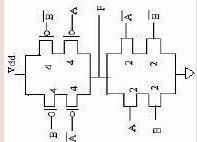Fig 22.4: XOR Gate

Here we will calculate the logical effort for a bundle (A* or B*) instead of only one input as complementary inputs are applied.

Logical effort for a bundle A is g = (2+4+2+4)/ (1+2) = 4.
Logical effort for a bundle B is g = (2+4+2+4)/ (1+2) = 4.

22.6 Examples Circuits

Example Circuit 1: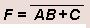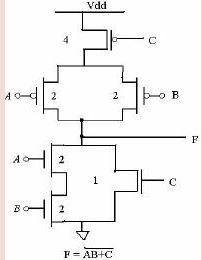Fig 22.61: Example Circuit 1

Example 1: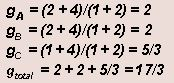Example2:

Logical effort for input D is
gD = (2+4)/ (1+2) = 2
Logical effort for bundle S is
gs =(2+4)/ (1+2) = 2.
For one arm, g = 12/3 = 4
For N-way symmetrical MUX
g= 4N (this is for the static CMOS MUX only)

Example Circuit 2: 4 BIT MUX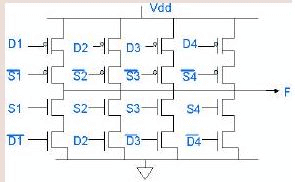Fig 22.62: Example Circuit 2

22.7 Tabular View of Logical Efforts

Logical effort for different circuits is tabulated in the table below in fig. 22.71Fig 22.71: Logical efforts of basic gates with different input configurations

The parasitic delays for different is tabulated in fig.  22.72.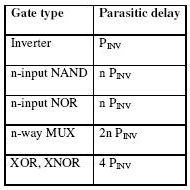Fig 22.72: parasitic delay of basic gates

Now delay,
d = gh+ p
For example, dINV = (1*1) +1=2.
If we assume
tau = 25 ps
Absolute delay dABS =50 ps.

22.8 Delay Plot

The delay of a simple logic gate as represented in equation d = gh + p is a simple linear relationship. The fig 22.8 shows this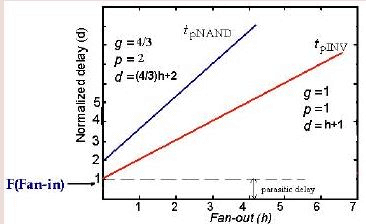Fig 22.8: Delay Plot

relationship graphically. Delay appears as a function of electrical effort for an inverter and for a two-input NAND gate.The slope of each line is the logical effort of the gate.It’s intercept is the parasitic delay. The graph shows that we can adjust the total delay by adjusting the electrical effort or by choosing a logic gate with a different logical effort.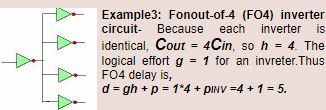Fig22.82: FO4 circuit

Offer running on EduRev: Apply code STAYHOME200 to get INR 200 off on our premium plan EduRev Infinity!

,

,

,

,

,

,

,

,

,

,

,

,

,

,

,

,

,

,

,

,

,

;